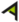# What is the difference between Vertical Angles and Angle of Depression?

In mathematics, the angle of depression and vertical angle are two different concepts. But both are critical points in trigonometry and help to do calculations of unknown angles, sides, and distances.

In this article, you will learn the difference between the angle of depression and the vertical angle. But before learning their difference, let’s discuss what vertical angles are.

## What are Vertical Angles?

When two lines cross at a place in such a way that four angles are generated. These angles are directly opposed and measure the same. These four angles are known as vertical angles if they are equal and opposing to each other without sharing any sides.

Vertical angles are always congruent, which means that they are equal in measure and oppose each other. For example, when two lines AB and EF intersect each other at their common point O, such that:

The measure of angle between A and E is equal to the measure of angle between B and F.

These four angles in the above figure, which are equal to their adjacent angles, known as vertical angles. Vertical angle calculator helps you to find the adjacent angles. And these angles are calculated by using congruent and supplementary theorems.

## What is the angle of depression?

The angle of depression is an angle between the horizontal line and the light of sight of an observer. When you look down at an object, this angle is formed between your line of sight and the horizontal distance between you and the object.

In trigonometry, the angle of depression measures an unknown height or distance between two objects. The horizontal line may refer to the base, and the height may refer to the perpendicular of a triangle. Therefore, it is used to solve many triangular problems.

Angle of depression calculator works fine to calculate angle of depression using the trigonometric function tangent, which is a triangle’s ratio of base and hypotenuse. The formula to calculate the angle between the line of sight of the observer and the object is as follows:

Up to 30% off on a selection of models at Lenovo!

### Lenovo 300 Series

Ideapad 300 Series laptops – lightweight and highly reliable for on-the-go productivity.

Enhanced graphics, multiple screen sizes & speedy processing

Starting at: \$332.99

## Difference between angle of depression and vertical angle

The angle of depression and vertical angle are totally different from each other. But they have many applications in mathematics along with engineering and science. The major differences between angle of depression and vertical angles are given in the following difference table.

## Conclusion

The vertical angles and angles of depression are two completely different concepts but we have one online calculator for both of them.

But they follow the same congruence theorem such that the angle of depression and elevation are congruent. And vertical angles are also congruent. The angle of depression is formed between the line of sight and the distance between the object.

At the same time, the vertical angles are created by the intersection of two straight lines at a common point. But both concepts are essential in trigonometry, which helps find the unknown angle of the side of a triangle.

Lenovo

### IdeaPad Gaming 3i (15”) laptop

• Premium 10th Generation Intel® Core™ processors
• Get up to 2x performance with NVIDIA® GeForce® GTX 1650 graphics
• 15.6” FHD IPS display, up to 120Hz
• Features a more robust dual fan and vent system than previous gens & offers users control of their fan speed
• Take advantage of a limited time low monthly price on Xbox Game Pass for PC

## FAQ’s

### What is the angle of the depression formula?

The angle of depression formula is used to calculate the unknown angle or side of a triangle. This formula is:

Where, the horizontal is the distance between the line of sight and the object.

### Do vertical angles equal 90?

Yes, the vertical angles can be 90 degrees. Such angles can be formed when two straight lines intersect each other at right angles. According to the supplementary theorem, two neighbouring angles add up to 180 degrees.

### Anza Rectangle Navy Eyeglasses

Anza is a classic rectangular frame with a modern feel. Its pliable plastic frame can bend without breaking, making it the perfect travel companion for sleeping on planes or packing in luggage. Part of our Flexi Frames collection, designed to make everyday eyewear even more comfortable and convenient.

US\$45

### What is vertical and horizontal?

Vertical and horizontal are two terms that describe direction. Horizontal describes the direction across the ground and the vertical line describes upward direction. These vertical and horizontal lines make a coordinate system together.

### Are vertical angles perpendicular?

The vertical angles are always congruent. They can be perpendicular if two straight lines intersect each other at right angles. The axis of the plane (xy-coordinates) is an example of perpendicular lines.

______________________________________

### Every month in 2023 we will be giving away one PlayStation 5. To qualify join our Facebook group,TikTok and Subscribe to our Sweet TnT Magazine YouTube channel

When you buy something through our retail links, we may earn commission and the retailer may receive certain auditable data for accounting purposes.

You may also like:

Lulus

### Garden Bliss Dusty Lavender Cutout Maxi Dress

Lulus Exclusive! Get swept off your feet in the Lulus Garden Bliss Dusty Lavender Cutout Maxi Dress! Elegant chiffon shapes this stunning dress featuring a rounded neckline, short fluttery sleeves, and a darted bodice. High fitted waist tops a cascading maxi skirt with a stunning side slit, while a back cutout adds a little something extra that’s sure to turn heads.

US\$89.00